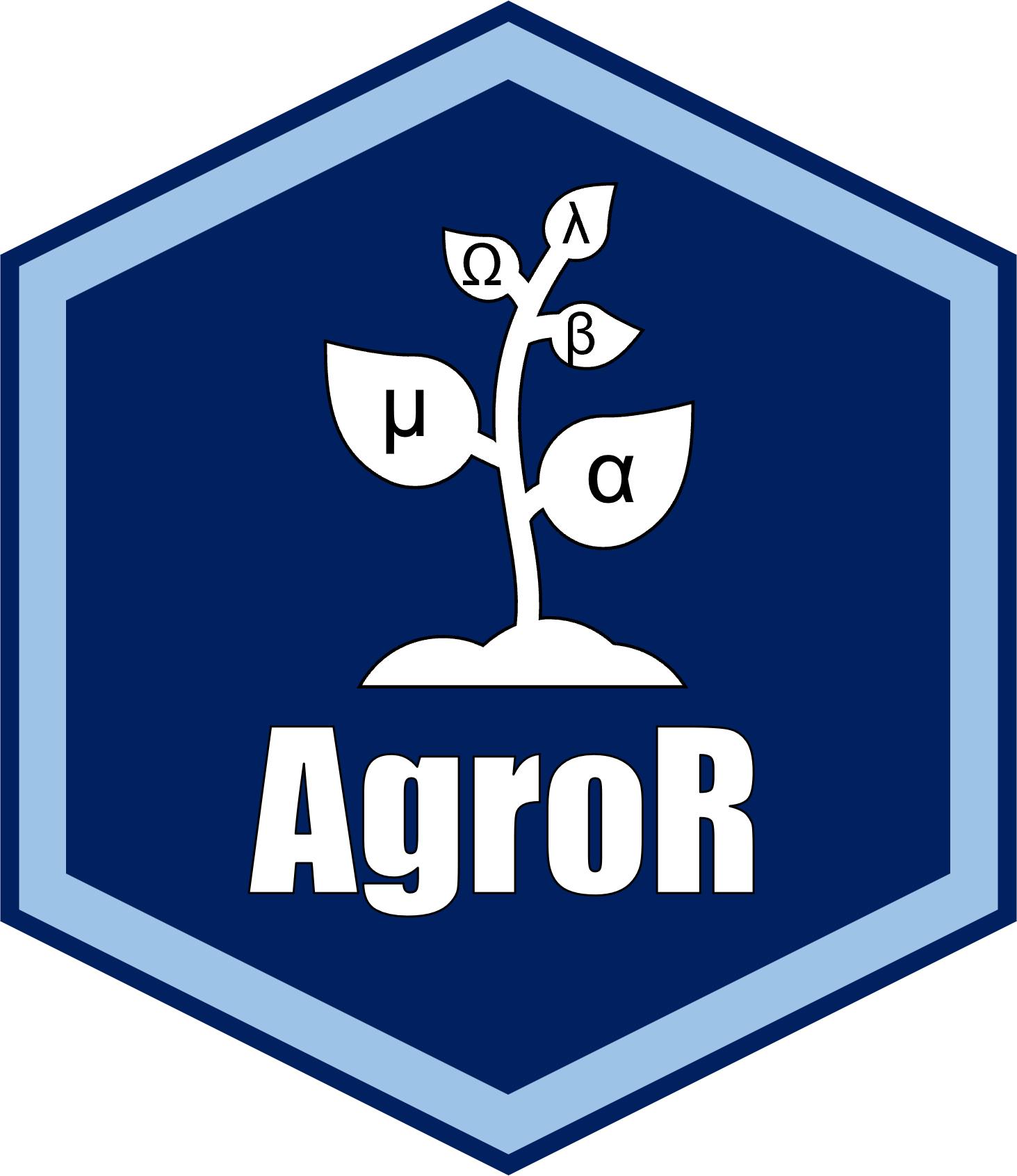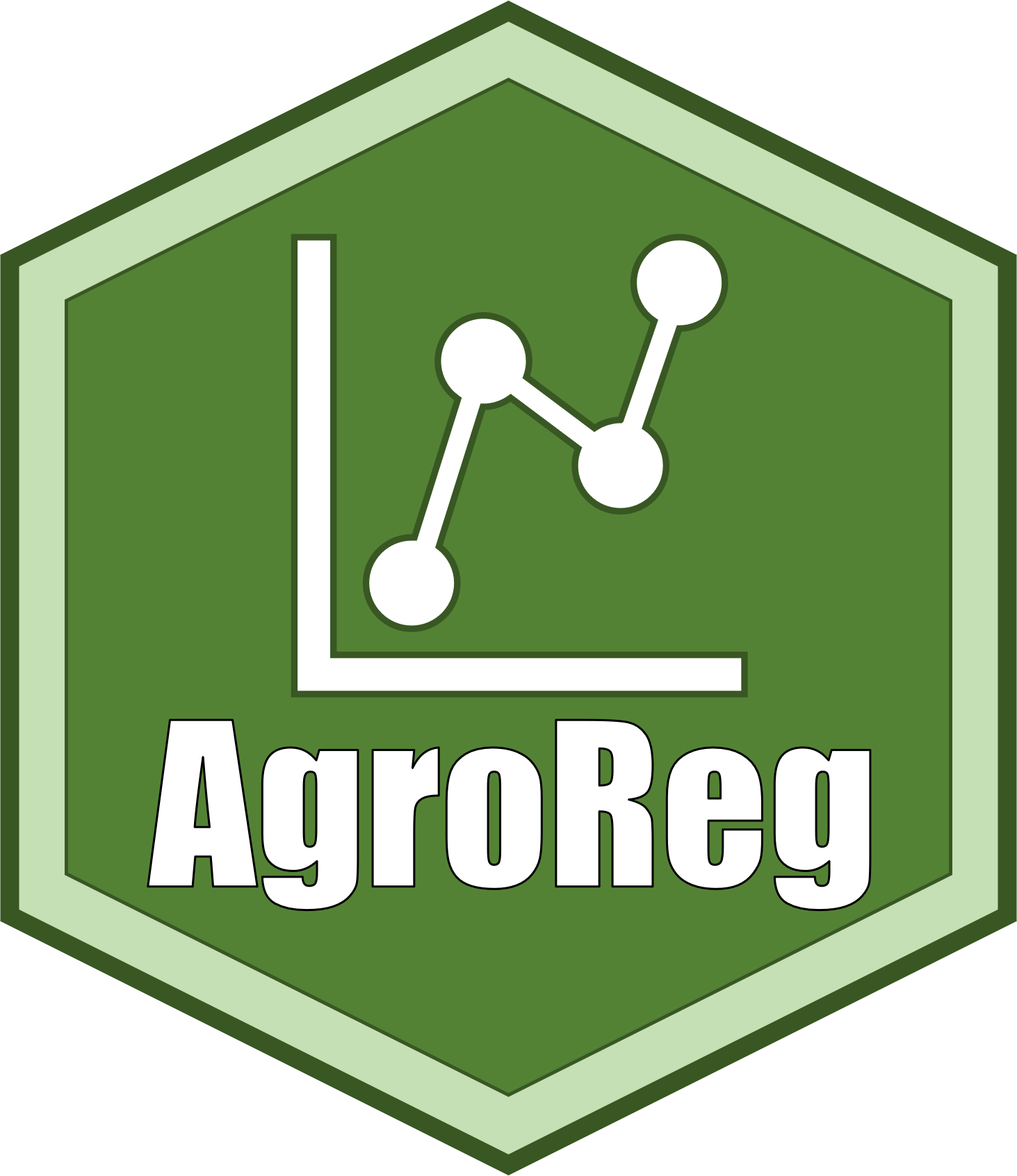## AgroR Shiny App## AgroReg Shiny App## AgroMulti Shiny App## R for education## Pacotes RPerforms the analysis of completely randomized experimental designs (CRD), randomized blocks (RBD) and Latin square (LSD), experiments in double and triple factorial scheme (in CRD and RBD), experiments in subdivided plot scheme (in CRD and RBD), subdivided and joint analysis of experiments in CRD and RBD, linear regression analysis, test for two samples. The package performs analysis of variance, ANOVA assumptions and multiple comparison test of means or regression, nonparametric test, test for two samples, joint analysis of experiments and generalized linear model (glm) for binomial and Poisson family in CRD and RBD.Linear and nonlinear regression analysis common in agricultural science articles. The package includes polynomial, exponential, gaussian, logistic, logarithmic, segmented, non-parametric models, among others. The functions return the model coefficients and their respective p values, coefficient of determination, root mean square error, AIC, BIC, as well as graphs with the equations automatically.The package is dedicated to regression analysis of common models in scientific works and others with potential for use in estimating the optimal, maximum and minimum temperature for the occurrence of seed germination.

### Desenvolvedores:Professor de estatística, mestre em agronomia, pós-graduado em estatística quantitativaProfessor de melhoramento genético, mestre e doutor em agronomiaProfessor de mecanização agrícola e estatística, mestre e doutor em agronomia

#### Apoio:

ATI – Assessoria de Tecnologia de Informação - UEL
Departamento de Agronomia - UEL
Departamento de estatítica - UEL
Programa de Pós-graduação em Agronomia - UEL
Em desenvolvimento
Testando
Testando
Testando